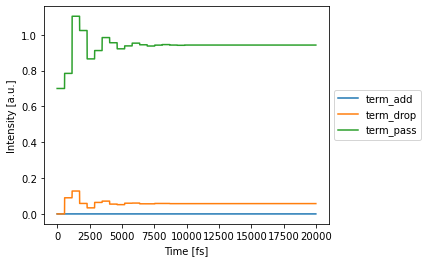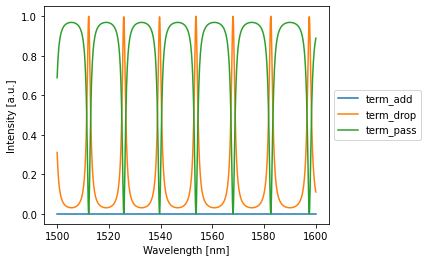# General Ring Networks¶

## Imports¶

:

import torch
import numpy as np
import matplotlib.pyplot as plt
import photontorch as pt
from tqdm.notebook import trange

torch.manual_seed(42)
np.random.seed(33)


## Add Drop Filter¶

Let’s try to recreate the add drop filter in 02_add_drop.ipynb, but this time using the RingNetwork class:

:

env = pt.Environment(
dt = 1e-14,
t_end=2000e-14,
wl=1e-6*np.linspace(1.5, 1.6, 1000),
)
env

:

key value description
nameenvname of the environment
t[0.000e+00, 1.000e-14, ..., 1.998e-11][s] full 1D time array.
t00.000e+00[s] starting time of the simulation.
t11.999e-11[s] ending time of the simulation.
num_t1999number of timesteps in the simulation.
dt1.000e-14[s] timestep of the simulation
samplerate1.000e+14[1/s] samplerate of the simulation.
bitrateNone[1/s] bitrate of the signal.
bitlengthNone[s] bitlength of the signal.
wl[1.500e-06, 1.500e-06, ..., 1.600e-06][m] full 1D wavelength array.
wl01.500e-06[m] start of wavelength range.
wl11.600e-06[m] end of wavelength range.
num_wl1000number of independent wavelengths in the simulation
dwl1.001e-10[m] wavelength step sizebetween wl0 and wl1.
f[1.999e+14, 1.998e+14, ..., 1.874e+14][1/s] full 1D frequency array.
f01.999e+14[1/s] start of frequency range.
f11.874e+14[1/s] end of frequency range.
num_f1000number of independent frequencies in the simulation
df-1.334e+10[1/s] frequency step between f0 and f1.
c2.998e+08[m/s] speed of light used during simulations.
freqdomainFalseonly do frequency domain calculations.
:

nw = pt.RingNetwork(
N=2,
capacity = 3,
wg_factory = lambda: pt.Waveguide(length=50e-6/2, neff=2.86),
mzi_factory = lambda: pt.DirectionalCoupler(coupling=0.3),
).terminate([pt.Source("term_in"), pt.Detector("term_pass"), pt.Detector("term_drop"), pt.Detector("term_add")])
with env.copy(wl=env.wavelength.mean()):
detected = nw(source=1)
nw.plot(detected)
plt.show()

with env.copy(freqdomain=True):
detected = nw(source=1)
nw.plot(detected)
plt.show()Of course you can create much bigger and complicated ring networks, but that’s for another time.